Are you searching for the Samacheer Kalvi 12th Chemistry Chapter Wise Solutions PDF? Then, get your Samacheer Kalvi 12th Chapter Wise Solutions PDF for free on our website. Students can Download Chemistry Chapter 1 Metallurgy Questions and Answers, Notes Pdf, Samacheer Kalvi 12th Chemistry Solutions Guide Pdf helps you to revise the complete Tamilnadu State Board New Syllabus and score more marks in your examinations.

## Tamilnadu Samacheer Kalvi 12th Chemistry Solutions Chapter 1 Metallurgy

All concepts are explained in an easy way on our website. So, students can easily learn and practice Tamilnadu State Board 12th Chemistry Chapter 1 Metallurgy Question and Answers. You can enjoy the digitized learning with the help of the Tamilnadu State Board Chemistry Online Material.

### Samacheer Kalvi 12th Chemistry Metallurgy TextBook Evalution

12th Chemistry Chapter 1 Book Back Answers Question 1.
Bauxite has the composition ………………
(a) Al2O3
(b) Al2O3.nH2O
(c) Fe2O3.2H2O
(d) None of these
(b) Al2O3.nH2O

Metallurgy Class 12 State Board Question 2.
Roasting of sulphide ore gives the gas (A). (A) is a colourless gas. Aqueous solution of (A) is acidic. The gas (A) is ………………
(a) CO2
(b) SO3
(c) SO2
(d) H,S
(c) SO2

Metallurgy Class 12 Important Questions State Board Question 3.
Which one of the following reaction represents calcination?
(a) 2Zn + O2 → 2ZnO
(b) 2ZnS + 3O2 → 2ZnO + 2SO2
(c) MgCO3 → MgO + CO2
(d) Both (a) and (c)
(c) MgCO3 → MgO + CO2

Samacheer Kalvi Guru 12th Chemistry Question 4.
The metal oxide which cannot be reduced to metal by carbon is ………………
(a) PbO
(b) Al2O3
(C) ZnO
(d) FeO
(b) Al2O3

12th Chemistry Samacheer Kalvi Question 5.
Which of the metal is extracted by Hall-Herold process?
(a) Al
(b) Ni
(c) Cu
(d) Zn
(a) Al

12th Chemistry Metallurgy Book Back Answers Question 6.
Which of the following statements, about the advantage of roasting of sulphide ore before reduction is not true?
(a) ∆Gf° of sulphide is greater than those for CS2 and H2S.
(b) ∆Gr° is negative for roasting of sulphide ore to oxide.
(c) Roasting of the sulphide to its oxide is thermodynamically feasible.
(d) Carbon and hydrogen are suitable reducing agents for metal sulphides.
(d) Carbon and hydrogen are suitable reducing agents for metal sulphides.

12th Chemistry Metallurgy Pdf Question 7.
Match items in column -1 with the items of column – II and assign the correct code: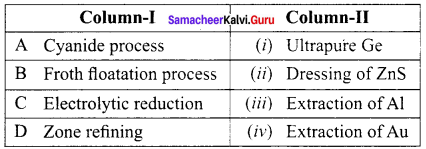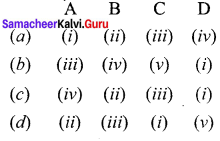(c) A – (iv), B – (ii), C – (iii), D – (i)

Chemistry Class 12 Samacheer Kalvi Question 8.
Wolframite ore is separated from tinstone by the process of ………………
(a) Smelting
(b) Calcination
(c) Roasting
(d) Electromagnetic separation
(d) Electromagnetic separation

12th Chemistry Metallurgy Question 9.
Which one of the following is not feasible?
(a) Zn(s) + Cu2+(aq) → Cu(s) + Zn2+(aq)
(b) Cu(s) + Zn2++(aq) → Zn(s) + Cu2+(aq)
(c) Cu(s) + 2Ag+(aq) → Ag(s) + Cu2+(aq)
(d) Fe(s) + Cu2+(aq) → Cu(s) + Fe2+(aq)
(b) Cu(s) + Zn2++(aq) → Zn(s) + Cu2+(aq)

Samacheer Kalvi 12 Chemistry Solutions Question 10.
Electrochemical process is used to extract ………………
(a) Iron
(c) Sodium
(d) Silver
(c) Sodium

12 Chemistry Samacheer Kalvi Question 11.
Flux is a substance which is used to convert ………………
(a) Mineral into silicate
(b) Infusible impurities to soluble impurities
(c) Soluble impurities to infusible impurities
(d) All of these
(b) Infusible impurities to soluble impurities

12th Chemistry Metallurgy Important Questions Question 12.
Which one of the following ores is best concentrated by froth – floatation method?
(a) Magnetite
(b) Hematite
(c) Galena
(d) Cassiterite
(c) Galena

Samacheer Kalvi 12th Chemistry Solutions Chapter 1 Question 13.
In the extraction of aluminium from alumina by electrolysis, cryolite is added to ………………
(a) Lower the melting point of alumina
(b) Remove impurities from alumina
(c) Decrease the electrical conductivity
(d) Increase the rate of reduction
(a) Lower the melting point of alumina

Zinc is obtained from ZnO by ………………
(a) Carbon reduction
(b) Reduction using silver
(c) Electrochemical process
(d) Acid leaching
(a) Carbon reduction

12th Samacheer Kalvi Chemistry Question 15.
Cupellation is a process used for the refining of ………………
(a) Silver
(c) Copper
(d) Iron
(a) Silver

Samacheer Kalvi 12th Chemistry Question 16.
Extraction of gold and silver involves leaching with cyanide ion. Silver is later recovered by ………………
(a) Distillation
(b) Zone refining
(c) Displacement with zinc
(d) liquation
(c) Displacement with zinc

Samacheer Kalvi Guru 12 Chemistry Question 17.
Considering Ellingham diagram, which of the following metals can be used to reduce alumina?
(a) Fe
(b) Cu
(c) Mg
(d) Zn
(c) Mg

Samacheer Kalvi 12th Chemistry Book Solutions Question 18.
The following set of reactions are used in refining Zirconium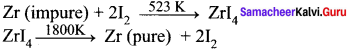This method is known as
(a) Liquation
(b) Van Arkel process
(c) Zone refining
(d) Monds process
(b) Van Arkel process

12th Chemistry Solution Book Samacheer Kalvi Question 19.
Which of the following is used for concentrating ore in metallurgy?
(a) Leaching
(b) Roasting
(c) Froth floatation
(d) Both (a) and (c)
(d) Both (a) and (c)

Class 12 Chemistry Chapter 1 Notes Question 20.
The incorrect statement among the following is ………………
(a) Nickel is refined by Monds process
(b) Titanium is refined by Van Arkels process
(c) ZinC blende is concentrated by froth floatation
(d) In the metallurgy of gold, the metal is leached with dilute sodium chloride solution
(d) In the metallurgy of gold, the metal is leached with dilute sodium chloride solution

12th Chemistry 1 Chapter Question 21.
In the electrolytic refining of copper, which one of the following is used as anode?
(a) Pure copper
(b) Impure copper
(c) Carbon rod
(d) Platinum electrode
(b) Impure copper

Metallurgy Class 12 Important Questions Pdf Question 22.
Which of the following plot gives Ellingham diagram?
(a) ∆S Vs T
(b) ∆G° Vs T
(c) ∆G° Vs
(d) ∆G° Vs T
(b) ∆G° Vs T

Samacheer Kalvi 12th Chemistry Solutions Question 23.
In the Ellingham diagram, for the formation of carbon monoxide
(a) $$\left( \frac { \triangle { S }^{ 0 } }{ \triangle T } \right)$$is negative
(b) $$\left( \frac { \triangle { G }^{ 0 } }{ \triangle T } \right)$$is positive
(c) $$\left( \frac { \triangle { G }^{ 0 } }{ \triangle T } \right)$$is negative
(d) initially $$\left( \frac { \triangle T }{ \triangle { G }^{ 0 } } \right)$$is positive, after 700°C, $$\left( \frac { \triangle { G }^{ 0 } }{ \triangle T } \right)$$is negative
(c) $$\left( \frac { \triangle { G }^{ 0 } }{ \triangle T } \right)$$is negative

Question 24.
Which of the following reduction is not thermodynamically feasible?
(a) Cr2O3 → Al2O3 + 2Cr
(b) Al2O3 → Cr2O3 + 2Al
(c) 3TiO2 + 4Al → 2Al2O3 + 2Al
(d) none of these
(b) Al2O3 → Cr2O3 + 2Al

Question 25.
Which of the following is not truc with respect to Ellingham diagram?
(a) Free energy changes follow a straight line. Deviation occurs when there is a phase change.
(b) The graph for the formation of CO2 is a straight line almost parallel to free energy axis.
(c) Negative slope of CO shows that it becomes more stable with increase in temperature.
(d) Positive slope of metal oxides shows that their stabilities decrease with increase in temperature.
(b) The graph for the fonnation of CO2 is a straight line almost parallel to free energy axis.

Question 1.
What is the difference between minerals and ores?
Minerals:

1. Minerals contain a low percentage of metal.
2. Metal cannot be extracted easily from minerals.
3. Clay Al2O3. SiO2. 2H2O is the mineral of aluminium.

Ores:

1. Ores contain a large percentage of metal.
2. Ores can be used for the extraction of metals on a large scale readily and economically.
3. Bauxite Al2O3. 2H2O is the ore of aluminium.

Question 2.
What are the various steps involved in extraction of pure metals from their ores?
The extraction of pure metals from the concentrated ores is carried out in two steps:

1. Conversion of the ore into oxides of the metal of interest.
2. Reduction of the metal oxides to elemental metals.

Question 3.
What is the role of Limestone in the extraction of Iron from its oxide Fe2O3?
In the extraction of iron, a basic flux limestone is used. Limestone decomposes to form CaO which reacts with silica gangue present in the iron ore is acidic in nature to form calcium silicate (slag).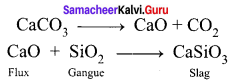Question 4.
Which type of ores can be concentrated by froth floatation method? Give two examples for such ores.
Sulphide ores can be concentrated by froth floatation method, e.g.,

1. Copper pyrites (CuFeS2H2)
2. Zinc blende (ZnS)
3. Galena (PbS)

Question 5.
Out of coke and CO, which is better reducing agent for the reduction of ZnO? Why?
Coke (C) is a better reducing agent for the reduction of ZnO. Because, when we use coke, the reduction can be easily carried out at 673 K. Thus Carbon (Coke) reduces zinc oxide more easily than carbon monoxide (CO). From the Ellingham diagrams, it is quite clear that the reduction of zinc oxide is more favourable using coke ∆G for the formation of carbon monoxide from carbon is more negative).

Question 6.
Describe a method for refining nickel.
The impure nickel is heated in a stream of carbon monoxide at around 350K. The nickel reacts with the CO to form a highly volatile nickel tetracarbonyl. The solid impurities are left behind.
Ni (s) + 4 CO (g) → Ni(CO)4(g)

On heating the nickel tetracarbonyl around 460 K, the complex decomposes to give pure metal.
Ni(CO)4 (g) → Ni (s) + 4 CO (g)

Question 7.
Explain zone refining process with an example using the Ellingham diagram given below.
1. Zone Refining method is based on the principles of fractional crystallisation. When an impure metal is melted and allowed to solidify, the impurities will prefer to be in the molten region, i.e. impurities are more soluble in the melt than in the solid state metal.

2. In this process, the impure metal is taken in the form of a rod. One end of the rod is heated using a mobile induction heater which results in melting of the metal on that portion of the rod.

3. When the heater is slowly moved to the other end the pure metal crystallises while the impurities will move on to the adjacent molten zone formed due to the movement of the heater. As the heater moves further away, the molten zone containing impurities also moves along with it.

4. The process is repeated several times by moving the heater in the same direction again and again to achieve the desired purity level.

5. This process is carried out in an inert gas atmosphere to prevent the oxidation of metals.

6. Elements such as germanium (Ge), silicon (Si) and galium (Ga) that are used as semiconductor are refined using this process.

Question 8.
1. Predict the conditions under which
(a) Aluminium might be expected to reduce magnesia.
(b) Magnesium could reduce alumina.

2. Carbon monoxide is more effective reducing agent than carbon below 983 K but, above this temperature, the reverse is true -Explain.

3. it is possible to reduce Fe2O3 by coke at a temperature around 1200 K.
1. The conditions under which:
(a) Ellingham diagram is used to predict thermodynamic feasibility of reduction of oxides of one metal by another metal. Any metal can reduce the oxides of other metals that are located above it in the Ellingham diagram. In the Ellingham diagram, for the formation of magnesia (magnesium oxide) occupy lower position than aluminium oxide. Therefore aluminium cannot be used to reduce the oxides of magnesium (magnesia). Above 1623K, A1 can reduce MgO to Mg, so that ArG° becomes negative and the process becomes thermodynamically feasible.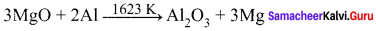(b)

• $$\left( \frac { 4 }{ 3 } \right)$$Al + O2 → $$\left( \frac { 2 }{ 3 } \right)$$Al2O3
• 2Mg + O2 → 2MgO

At the point of intersection of the Al2O3 and MgO curves in Ellingham diagram. ∆G°
becomes zero for the reaction:

$$\left( \frac { 2 }{ 3 } \right)$$Al2O3 → 2MgO + $$\left( \frac { 4 }{ 3 } \right)$$Al
Below that point magnesium can reduce alumina.

2. From the Ellingham diagram, we find that at 983 K, the curves intersect.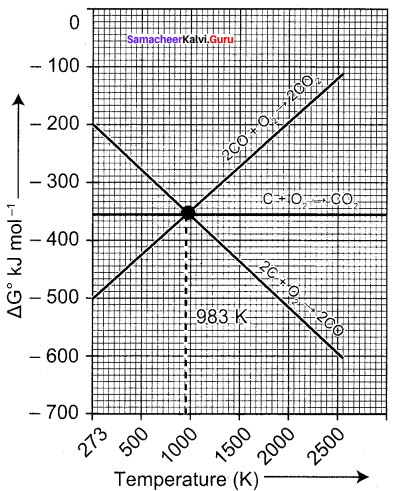The value of ∆G° for change of C to CO2 is less than the value of ∆G° for change of CO to CO2. Therefore, coke (C) is a better reducing agent than CO at 983K or above temperature. However below this temperature (e.g. at 673K), CO is more effective reducing agent than C.

3. Yes, it is possible to reduce Fe2O3 by coke at a temperature around 1200 K. In the Ellingham diagram, carbon line cuts across the lines of many metal oxides and hence it can reduce all those metal oxides at sufficiently high temperature. Ellingham diagram for the formation of Fe2O3 and CO intersects around 1000 K.

Below this temperature, the carbon line lies above the iron line which indicates that Fe2O3 is more stable than CO and hence at this temperature range the reduction is not thermodynamically feasible. However above 1000 K carbon line lies below the iron line and hence we can use coke as a reducing agent around 1200 K. Around 1200 K, coke is better reducing agent because above 1000 K, Gibb’s free energy for the formation of Fe2O3 is more than the formation of CO2 from C.

Question 9.
Give the uses of zinc.
Applications of Zinc (Zn):

1. Metallic zinc is used in galvanising metals such as iron and steel structures to protect them from rusting and corrosion.
2. Zinc is also used to produce die-castings in the automobile, electrical and hardware industries.
3. Zinc oxide is used in the manufacture of many products such as paints, rubber, cosmetics, pharmaceuticals, plastics, inks, batteries, textiles arid electrical equipment. Zinc sulphide is used in making luminous paints, fluorescent lights and x-ray screens.
4. Brass an alloy of zinc is used in water valves and communication equipment as it is highly resistant to corrosion.

Question 10.
Explain the electrometallurgy of aluminium.
Electrochemical extraction of aluminium Hall-Herold process:
In this method, electrolysis is carried out in an iron tank lined with carbon, which acts as a cathode. The carbon blocks immersed in the electrolyte acts as a anode. A 20% solution of alumina, obtained from the . bauxite ore is mixed with molten cyrolite and is taken in the electrolysis chamber. About 10%, calcium chloride is also added to the solution. Here calcium chloride helps to lower the melting point of the mixture. The fused mixture is maintained at a temperature of above 1270 K. The chemical reactions involved in this process are as follows:

Ionisation of alumina:
Al2O3 → 2Al3 + 3OO2-

Reaction at cathode:
2Al3+ (melt) + 3e → Al(l)

Reaction at anode:
2O2- (melt) → O2 + 3e

Since carbon acts as anode the following reaction also takes place on it.

• C (s) + O2- (melt) → CO + 2e
• C (s) + 2O2- (melt) → CO2 + 4e

Due to the above two reactions, anodes are slowly consumed during the electrolysis. The pure aluminium is formed at the cathode and settles at the bottom. The net electrolysis reaction can be written as follows:
4Al3+ (melt) + 6O2- (melt) + 3C(s) → 4A(l) + 3CO2(g)

Question 11.
Explain the following terms with suitable examples.

1. Gangue
2. Slag

1. Gangue:
The impurities associated with the minerals are known as Gangue or Matrix.

2. Slag:
A compound formed when gangue is combined with flux is called slag.
Flux + Gangue → Slag

For example, the oxide of iron can be reduced by carbon monoxide as follows:
Fe2O3 + 3CO → 2Fe + 3CO2

In this extraction a basic flux, limestone is used.
Since the silica gangue present in the ore is acidic in nature, the limestone combines with it to form Calcium silicate (Slag).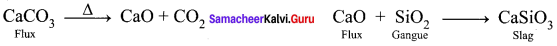Question 12.
Give the basic requirement for vapour phase refining.
The two requirements for vapour phase refining are:

1. The metal should form a volatile compound with a suitable reagent.
2. The volatile compound is decomposed to give the pure metal.

Question 13.
Describe the role of the following in the process mentioned.

1. Silica in the extraction of copper.
2. Cryolite in the extraction of aluminium.
3. Iodine in the refining of Zirconium.
4. Sodium cyanide in froth floatation.

1. The role of silica in the extraction of copper is to remove the iron oxide obtained during the process of roasting as slag. If the sulphide ore of copper contains iron, the silica (SiO2) is added as flux before roasting. Then, FeO combines with silica to form iron silicate, FeSiO3 (Slag).

2. Cryolite reduces the melting point of Al2O3 and increases its electrical conductivity. Aluminium is produced by the electrolytic reduction of fused alumina in the electrolytic cell. Alumina is not an electrolyte. So it is made as an electrolyte by dissolving it in the fused cryolite. The function of cryolite is to lower the fusion temperature.

3. Zirconium crude metal is heated with iodine in an evacuated vapour to separate from impurities and this decomposes at 1800 K to give a pure zirconium metal and iodine. Initially iodine is heated with zirconium to form a volatile compound.

4. Sulphide ores which are concentrated by the froth floatation process. Depressants are used to prevent certain type of particles from forming the froth. NaCN act as a depressant to separate ZnS from PbS.

Question 14.
Explain the principle of electrolytic refining with an example.
The crude metal is refined by electrolysis. It is carried out in an electrolytic cell containing aqueous solution of the salts of the metal of interest. The rods of impure metal are used as anode and thin strips of pure metal are used as cathode.

The metal of interest dissolves from the anode, pass into the solution while the same amount of metal ions from the solution will be deposited at the cathode. During electrolysis, the less electropositive impurities in the anode, settle down at the bottom and are removed as anode mud. Let us understand this process by considering electrolytic refining of silver as an example.

Cathode:
Pure silver

Anode:
Impure silver rods

Electrolyte:
Acidified aqueous solution of silver nitrate. When a current is passed through the electrodes the following reactions will take place Reaction at anode.
2Ag (s) → Ag+(aq) + 1 e

Reaction at cathode:
Ag+ (aq) + 1 e → Ag (s)

During electrolysis, at the anode the silver atoms lose electrons and enter the solution. The positively charged silver cations migrate towards the cathode and get discharged by gaining electrons and deposited on the cathode. Other metals such as copper, zinc etc.,can also be refined by this process in a similar manner.

Question 15.
The selection of reducing agent depends on the thermodynamic factor. Explain with an example.
From the Ellingham diagram, it is clear that metals for which the standard free energy of formation (∆fG0) of their oxides is more negative can reduce the metal oxides for which the standard free energy of formation (∆fG0) of oxides is less negative.

Thermodynamic factor has a major role in selecting the reducing agent for a particular reaction. Only that reagent will be preferred which will lead to decrease in the free energy (AG°) at a certain specific temperature.
E.g – Carbon reduce ZnO to Zn but not CO.

• ZnO + C → Zn + CO …………..(1)
• ZnO + CO → Zn + CO2 ………………(2)

In the first case, there is increase in the magnitude of ∆S° while in the second case, it almost remains the same. In other words, ∆G° will have more negative value in the first case, when C is the reducing agent then in the second case when CO acts as the reducing agent. Therefore, C is a better reducing agent.

Question 16.
Give the limitations of Ellingham diagram.
Limitations of Ellingham diagram:
1. Ellingham diagram is constructed based only on thermodynamic considerations. It gives information about the thermodynamic feasibility of a reaction. It does not tell anything about the rate of the reaction. Moreover, it does not give any idea about the possibility of other reactions that might be taking place.

2. The interpretation of ∆G is based on the assumption that the reactants are in equilibrium with the product which is not always true.

Question 17.
Write a short note on electrochemical principles of metallurgy.
Electrochemical principles also find applications in metallurgical process. The reduction of oxides of active metals such as sodium, potassium etc., by carbon is thermodynamically not feasible. Such metals are extracted from their ores by using electrochemical methods. In this technique, the metal salts are taken in a fused form or in solution form. The metal ion present can be reduced by treating it with some suitable reducing agent or by electrolysis. Gibbs free energy change for the electrolysis process is given by the following expression
∆G° = -nFE°

Where n is number of electrons involved in the reduction process, F is the Faraday and E° is the electrode potential of the redox couple. If E° is positive then the ∆G is negative and the reduction is spontaneous and hence a redox reaction is planned in such a way that the e.m.f of the net redox reaction is positive. When a more reactive metal is added to the solution containing the relatively less reactive metal ions, the more reactive metal will go into the solution. For example,

• Cu (s) + 2Ag+(s) → Cu2+ (aq) + 2Ag (s)
• Cu2+ (aq) + Zn (s) → Cu (s) + Zn+(aq)

Evaluate Yourself

Question 1.
Write the equation for the extraction of silver by leaching with sodium cyanide and show that the leaching process is a redox reaction.
The crushed ore of argentite (Ag2S) is leached with sodium cyanide solution. This reaction forms sodium argento cyanide
Na[Ag(CN)2]

Step 1:
Ag2S + 4NaCN $$\rightleftharpoons$$ 2Na[Ag(CN)2] + Na2S
The solution of sodium argento cyanide combines with zinc dust and forms sodium tetra cyano zincate and precipitated silver.

Step 2:
Zn + 2Na[Ag(CN)2] → Na2[Ag(CN)4] + 2 Ag↓
In the step 2, redox reaction take place.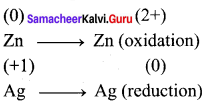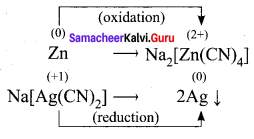Question 2.
Magnesite (Magnesium carbonate) is calcined to obtain magnesia, which is used to make refractory bricks. Write the decomposition reaction.
Magnesite is a carbonate of magnesium. Magnesite when heated at 800°C to 1000°C at the CO2 content in it is driven off. The residue so obtained is known as calcined magnesite.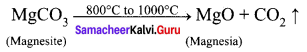Question 3.
Using Ellingham diagram indicate the lowest temperature at which ZnO can be reduced to zinc metal by carbon. Write the overall reduction reaction at this temperature.
Ellingham diagram shows variation in standard Gibbs free energy change with temperature for the formation of oxide. The Ellingham diagram shows straight line upward slope with formation of oxide, but in case of ZnO there is sudden change. Ellingham diagram helps in the selecting suitable reducing agent.

By seeing the Ellingham diagram, the free energy formation (∆fG°) of CO from C becomes lower temperatures above 1120 K while that of CO2 from C becomes lower above 1323 K than ∆fG° of ZnO. As ∆fG° of C02 from CO is always higher than that of ZnO. So C can reduce ZnO to Zn but not CO. Thus carbon is better reducing agent than CO for ZnO.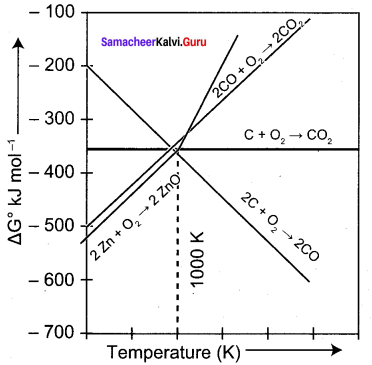Question 4.
Metallic sodium is extracted by the electrolysis of brine (aq. NaCl). After electrolysis the electrolytic solution becomes basic in nature. Write the possible electrode reactions.
Brine is a solution of sodium chloride (molten state):
The process of electrolysis involves using an electric current to bring about a chemical change and make new chemicals. In the electrolysis of brine, sodium ions migrate to the cathode, where electrons enter the melt and are reduced to sodium metal.
Na+ + e → Na (at cathode)

Chloride ions migrate the other way toward the anode. They give up their electrons to the anode and are oxidised to chlorine gas.
Cl → $$\frac { 1 }{ 2 }$$Cl2 + e (at anode)

Overall reaction:
2NaCl → 2Na(s) + Cl2 (g)

For aqueous solution of NaCl:

• H2O + 2e → H2$$\uparrow$$+ 20H (at cathode)
• Cl → $$\frac { 1 }{ 2 }$$Cl2 + e (at anode)

Overall reaction:
NaCl (aq) + H2O(1) → Na+(aq) + OH(aq) + H2(g) + $$\frac { 1 }{ 2 }$$Cl2(g)
After electrolysis the electrolytic solution becomes basic in nature. [Due to formation of hydroxide (OH) ion].

### Samacheer Kalvi 12th Chemistry Metallurgy Additional Questions

Samacheer Kalvi 12th Chemistry Metallurgy 1 Mark Questions and Answers

Question 1.
Chemical formula of bauxite is ……………
(a) Fe2O3
(b) Fe2O3. FeO
(c) Al2O3 . H2O
(d) Al2O3.2H2O
(d) Al2O3.2H2O

Question 2.
An example of an oxide ore is ……………
(a) malachite
(b) bauxite
(c) galena
(d) zinc blende
(b) bauxite

Question 3.
Froth floatation process involves the ……………
(a) treatment of the ore with water and pine oil
(b) washing of the ore with a steam of water
(c) owing off the ore over a conveyor belt rolling over magnetic roller
(d) melting of ore
(a) treatment of the ore with water and pine oil

Question 4.
In the froth floatation process for the purification of ores the particles float because ……………
(a) they are light
(b) their surface is not easily wetted by water
(c) they bear electrostatic charge
(d) they are insoluble
(b) their surface is not easily wetted by water

Question 5.
In a metallurgical process, an acid flux is used for removing ……………
(a) Slag
(b) basic flux
(c) acidic gangue
(d) basic gangue
(d) basic gangue

Question 6.
The process of the removal of impurities from a crude metal is called ……………
(a) concentration
(b) calcination
(c) refining
(d) roasting
(c) refining

Question 7.
Which of the following metal is obtained by self reduction method?
(a) Fe
(b) Cu
(c) Ag
(d) Mg
(b) Cu

Question 8.
Which one of the following ore is best concentrated by froth floatation method?
(a) Magnetite
(b) Malachite
(c) Galena
(d) Haematite
(c) Galena

Question 9.
Heating mixture of Cu2O and Cu2S will give ……………
(a) Cu + SO2
(b) Cu + SO3
(c) CuO + CuS
(d) Cu2SO3
(a) Cu + SO2

Question 10.
Which of the following pairs of metals is purified by Van-Arkel method?
(a) Ag and Au
(b) Ni and Fe
(c) Ga and In
(d) Zr and Ti
(d) Zr and Ti

Question 11.
Aluminium is extracted from alumina (Al2O3) by electrolysis of a molten mixture of ……………
(a) (Al2O3) + KF + Na3AlF6
(b) (Al2O3) + HF + NaAlF4
(c) (Al2O3) + CaF2 + NaAlF4
(d) (Al2O3) + Na3AlF6 + CaF2
(d) (Al2O3) + Na3AlF6 + CaF2

Question 12.
The ore which contains both copper and iron ……………
(a) Cuprite
(b) Haematite
(c) Copper pyrite
(d) Malachite
(c) Copper pyrite

Question 13.
Match the extraction processes listed in Column-I with metals listed in Column-II.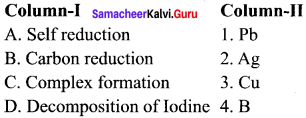(a) A – 2, B – 1, C – 4, D – 3
(b) A – 3, B – 4, C – 1, D – 2
(c) A – 3, B – 1, C – 2, D – 4
(d) A – 4, B – 2, C – l, D – 3
(c) A – 3, B – 1, C – 2, D – 4

Question 14.
Chief ore of aluminium is ……………
(a) bauxite
(b) clay
(c) haematite
(d) magnetite
(a) bauxite

Question 15.
Which one of the following metal having least chemical reactive?
(a) Na
(b) Mg
(c) Al
(d) Au
(d) Au

Question 16.
Pick out the more reactive metal.
(a) Cu
(b) Ag
(c) Au
(d) Na
(d) Na

Question 17.
Consider the following statements.
(i) All ores are minerals but all minerals are not ores.
(ii) Bauxite is an ore of aluminium while clay is not.
(iii) Extraction of aluminium form clay is profitable one.

Which of the above statement(s) is / are not correct?
(a) (i) only
(b) (ii) only
(c) (iii) only
(d) (i), (ii) and (iii)
(c) (iii) only

Question 18.
Match the List-I and List-II correctly using the code given below.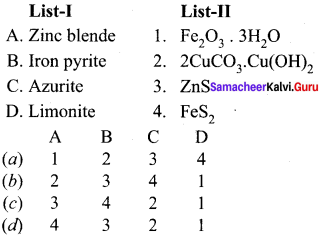(c)  3, 4, 2, 1

Question 19.
The impurities associated with ores is ……………
(a) flux
(b) slag
(c) gangue
(d) metal
(c) gangue

Question 20.
Oxide ores are concentrated by ……………
(a) handpicking
(b) hydraulic washing
(c) froth floatation process
(d) Magnetic separation process
(b) hydraulic washing

Question 21.
Haematite and tin stone are purified by ……………
(a) gravity separation process
(b) magnetic separation process
(c) froth floatation process
(d) handpicking
(a) gravity separation process

Question 22.
Froth floatation process is applicable for ……………
(a) oxide ores
(b) carbonate ores
(c) chloride ores
(d) sulphide ores
(d) sulphide ores

Question 23.
Copper pyrite and zinc blende are purified by ……………
(a) gravity separation process
(b) magnetic separation process
(c) froth floatation process
(c) handpicking
(c) froth floatation process

Question 24.
Frothing agent used in froth floatation process is ……………
(a) pine oil
(b) olive oil
(c) mustard oil
(d) neem oil
(a) pine oil

Question 25.
Depressing agents used to separate ZnS from PbS is ……………
(a) NaCN
(b) NaCl
(c) NaNO3
(d) NaNO2
(a) NaCN

Question 26.
Leaching is also called as ……………
(a) hand picking
(b) Electrolysis
(c) Chemical process
(d) magnetic separation process
(c) Chemical process

Question 27.
In the leaching process, the metal present in the ore is converted into ……………
(a) soluble salt
(b) soluble complex
(c) insoluble complex
(d) both (a) and (b)
(d) both (a) and (b)

Question 28.
Gold ore is concentrated by ……………
(a) cyanide leaching
(b) alkali leaching
(c) acid leaching
(d) hand picking
(a) cyanide leaching

Question 29.
Bauxite is purified by ……………
(a) cyanide leaching
(b) alkali leaching
(c) acid leaching
(d) hand picking
(b) alkali leaching

Question 30.
Which type of leaching process convert insoluble sulphide ores into soluble sulphates?
(a) cyanide leaching
(b) alkali leaching
(c) acid leaching
(d) hand picking
(c) acid leaching

Question 31.
A chemist involves mining process and he got tow ores mixed together. If one is tinstone and another one is chromite, which type of process will be used to separate that two ores?
(a) Leaching process
(b) Froth floatation process
(c) Zone refining process
(d) Magnetic separation process
(d) Magnetic separation process

Question 32.
The process in which the concentrated ore is strongly heated in the absence of air is called as ……………
(a) Roasting
(b) Calcination
(c) Smelting
(d) Leaching
(b) Calcination

Question 33.
A chemical substance that forms an easily fusible slag with gangue is called as ……………
(a) flux
(b) pure metal
(c) ore
(d) impure metal
(a) flux

Question 34.
Blistered copper is ……………
(a) 98% pure copper
(b) 96% pure copper
(c) 97% pure copper
(d) 88% pure copper
(a) 98% pure copper

Question 35.
Ignition mixture used in aluminothermic process is ……………
(a) Mg + BaO2
(b) MgO + BaO
(c) Al2O3 + Mg
(d) Al2O3 + BaO2
(a) Mg + BaO2

Question 36.
For spontaneous reaction, the change in free energy should be ……………
(a) positive
(b) negative
(c) zero
(d) neutral
(b) negative

Question 37.
The change in Gibbs free energy for a reaction is expressed by ……………
(a) ∆G = ∆H + T∆S
(b) ∆G = ∆H – TS
(c) G = H – TS
(d) ∆G = ∆H – T∆S
(d) ∆G = ∆H – T∆S

Question 38.
Relationship between ∆G° and Kp is ……………
(a) ∆G° = – RT In Kp
(b) ∆G° = – R In Kp
(c) ∆G° = – T In Kp
(d) ∆G° = RT In Kp
(a) ∆G° = – RT In Kp

Question 39.
Consider the following statements.
(i) Ellingham drawn on a plot by considering the temperature in the x-axis and the standard free energy change for the formation of metal oxide in y-axis.
(ii) The resultant plot is straight line.
(ii) In the Ellingham diagram, ∆H as slope and ∆S as y-intercept.

Which of the above statement(s) is / are not correct?
(a) (i) and (ii)
(b) (ii) and (iii)
(c) (ii) only
(d) (iii) only
(d) (iii) only

Question 40.
Which of the following oxides is unstable j at moderate temperature?
(a) Al2O3
(b) Cr2O3
(c) MgO
(d) HgO
(d) HgO

Question 41.
The oxides will decompose on heating even in the absence of a reducing agent is ……………
(a) Ag2O
(b) HgO
(c) MgO
(d) both (a) and (b)
(d) both (a) and (b)

Question 42.
Consider the following statements.
(i) Ellingham diagram gives information about the thermodynamic feasibility of a reaction.
(ii) It explains the rate of the reaction.
(iii) Below 1000 K temperature, FeO is more stable than CO.

Which of the above statements (s) is / are not correct?
(a) (i) and (ii)
(b) (ii) and (iii)
(c) (iii) only
(d) (ii) only
(d) (ii) only

Question 43.
Gibbs free energy change for the electrolysis process is expressed by ……………
(a) ∆G° = – nFE°
(b) ∆G° = nF
(c) ∆G° = – nE°
(d) ∆G° = nFE°
(a) ∆G° = – nFE°

Question 44.
The technique used to refining zinc and mercury is ……………
(a) Liquation
(b) Distillation
(c) Zone refining
(d) Van-Arkel method
(b) Distillation

Question 45.
Which of the following is not purified by zone refining process?
(a) Ge
(b) Si
(c) Ga
(d) Al
(d) Al

Question 46.
Nickel is purified by ……………
(a) Mond process
(b) Van-Arkel method
(c) Zone refining
(d) Electrolytic refining
(a) Mond process

Question 47.
Titanium is purified by ……………
(a) Mond process
(b) Van-Arkel method
(c) Zone refining
(d) Electrolytic refining
(b) Van-Arkel method

Question 48.
The metal used for galvanisation of iron is ……………
(a) Al
(b) Zn
(c) Cu
(d) Au
(b) Zn

Question 49.
Which metal alloy is used in design of aeroplane parts?
(a) Al
(b) Zn
(c) Cu
(d) Au
(a) Al

Question 50.
Which metal is used for making coins and ornaments along with gold and other metals?
(a) Zn
(b) Al
(c) Cu
(d) Fe
(c) Cu

II. Fill in the blanks:

1. Minerals that contains a high percentage of metal from which it can be extracted conveniently and economically are called ……………
2. …………… helps us to select a suitable reducing agent and appropriate temperature range for reduction.
3. If E° is positive, then the ∆G is ……………
4. Chemical formula of cuprite is ……………
5. Gravity separation is also called as ……………
6. …………… ore is purified by gravity separation process.
7. In froth floatation process …………… acts as a collector.
8. Sodium cyanide is added to depresses the floatation property of ZnS by forming a layer of ……………
9. The process of gold reduced to its elemental state is called ……………
10. Leaching process is a …………… reaction.
11. Magnesite is calcined to give ……………
12. …………… a chemical substance that forms an easily fusible slag with gangue.
13. Flux + Gangue → ……………
14. In the extraction of iron …………… is removed as slag.
15. In the extraction of copper …………… is removed as slag.
16. Cr2O3 can be reduced by …………… an process.
17. …………… is used as a reducing agent for the reduction of chromic oxide.
18. …………… is purified by zone refining.
19. In the mond process, impure nickel is heated with …………… compound.
20. …………… filament is used to decompose titanium tetraoxide.
21. …………… is the most abundant metal.
22. …………… is used in packing materials for food items.
23. …………… is used in the manufacture of paints, rubber and cosmetics.
24. …………… is the first metal used by the humans.
25. …………… is one of the expensive and precious metals.
26. …………… is used for increasing the efficiency of solar cells and also used as catalysts.
27. …………… ore is concentrated by froth floatation process.
28. Zinc blende is concentrated by …………… process.
29. Gold ore is leached by adding of ……………

1. Ores
2. Ellingham diagram
3. negative
4. Cu2O
5. Hydraulic washing
6. oxide
7. Sodium ethyl xanthate
8. Na2[Zn(CN)4] – Sodium negative
9. Cementation
10. Redox
11. Magnesia
12. Flux
13. slag
14. Calcium silicate (CaSiO3)
15. Ferrous silicate (FeSiO3)
16. Aluminothermic process
17. Aluminium
18. semi-conductior
19. carbon monoxide
20. Tungsten
21. Aluminium
22. Aluminium foils
23. Aluminium
24. Zinc oxide
25. Copper
26. Gold
27. Gold nanoparticle
28. Sulphide
29. Froth floatation
30. NaCN

III. Match the following:

Question 1.
(i) Haematite – (a) Fe3O4
(ii) Siderite – (b) Fe3O3
(iii) Iron pyrite – (c) Fe3O3
(iv) Magnetite – (d) FeS2
(i) – (b)
(ii) – (c)
(iii) – (d)
(iv) – (a)

Question 2.
(i) Copper glance – (a) CuCO3. Cu(OH)2
(ii) Malachite – (b) Cu2S
(iii) Copper pyrite – (c) 2CuCO3. Cu(OH)2
(iv) Azurite – (d) CuFeS2
(i) – (b)
(ii) – (a)
(iii) – (d)
(iv) – (c)

Question 3.
(i) Zinc blende – (a) Al203.2H2O
(ii) Bauxite – (b) ZnC03
(iii) Zincite – (c) ZnS
(iv) Calamine – (d) ZnO
(i) – (c)
(ii) – (a)
(iii) – (d)
(iv) – (b)

Question 4.
(i) Tin stone – (a) AgCl
(ii) Argentite – (b) Ag3SbS3
(iii) Ruby silver – (c) Ag2S
(iv) Horn silver – (d) SnO2
(i) – (d)
(ii) – (c)
(iii) – (b)
(iv) – (a)

Question 5.
(i) Oxide ore – (a) Zinc blende
(ii) Carbonate ore – (b) Horn silver
(Hi) Sulphide ore – (c) Haematite
(iv) Chloride ore – (d) Calamine
(i) – (c)
(ii) – (d)
(iii) – (a)
(iv) – (b)

Question 6.
(i) Tin stone – (a) Magnetic separation process
(ii) Copper pyrite – (b) Leaching process
(Hi) Bauxite – (c) Froth floatation process
(iv) Chromite – (d) Hydraulic washing process
(i) – (d)
(ii) – (c)
(iii) – (b)
(iv) – (a)

Question 7.
(i) Aluminium – (a) cosmetics
(ii) Zinc oxide – (b) gas pipelines
(iii) Iron – (c) making coins
(iv) Copper – (d) bicycle chains
(i) – (b)
(ii) – (a)
(iii) – (d)
(iv) – (c)

Question 8.
(i) Gold nanopaticle – (a)Cast iron
(ii) Cast iron – (b) Cooking vessels
(iii) Metallic zinc – (c) Solar cells
(iv) Aluminium – (d) Pump stoves
(i) – (c)
(ii) – (d)
(iii) – (a)
(iv) – (b)

IV. Assertion and reasons:

Note:
In the following questions a statement of assertion followed by a statement of reason is given. Choose the correct answer out of the following choices.
(a) A and R are correct, R explains A
(b) A and R are correct, R does not explains A
(c) A is correct but R is wrong
(d) A is wrong but R is correct

Question 1.
Assertion(A) – Clay is an ore of aluminium while bauxite is not.
Reason (R) – Aluminium can be economically extracted from bauxite not from clay.
(d) A is wrong but R is correct

Question 2.
Assertion(A) – Haematite and tin stone are concentrated by hydraulic washing process.
Reason (R) – Oxide ores are heavy and have high specific gravity.
(a) A and R are correct, R explains A .

Question 3.
Assertion(A) – Sulphide ores are concentrated by froth floatation process.
Reason (R) – Sulphide ores are preferentially wetted by oil can be separated from gangue.
(a) A and R are correct, R explains A

Question 4.
Assertion(A) – A suitable reducing agent is selected based on the thermodynamic consideration.
Reason (R) – The reduction of metal oxide with a given reducing agent can occur if the free energy change for the coupled reaction is positive.
(c) A is correct but R is wrong

Question 5.
Assertion(A) – Aluminium can be used as a reducing agent for the reduction of chromic oxide.
Reason (R) – In the Ellingham diagram, formation of chromium oxide lies above that of the aluminium, therefore Al2O3 is more stable than Cr2O3.
(a) A and R are correct, R explains A

Question 6.
Assertion(A) – Zone refining is based on the principles of fractional crystallisation,
Reason (R) – This process is carried out in an inert gas temperature.
(b) A and R are correct, R does not explain A

Question 7.
Assertion(A) – Aluminium is used in the j design of chemical reactors.
Reason (R) – Aluminium has high resistance to corrosion.
(a) A and R are correct, R explains A

V. Find the odd one out and give the reasons:

Question 1.
(a) Nickel
(b) Silicon
(c) Germanium
(d) Galium
(a) Nickel
Reason:
Si, Ge and Ga are purified by I zone refining while Ni is not.

Question 2.
(a) Copper
(b) Zinc
(c) Silver
(d) Galium
(d) Galium
Reason:
Cu, Zn and Ag are purified by electrolytic refining while Ga is not.

Question 3.
(a) ∆G = -ve
(b) ∆H = -ve
(c) ∆S = +ve
(d) ∆S = -ve
(d) ∆S = -ve

Reason:
∆G=-ve, ∆H=-ve, ∆S=+ve are the conditions for spontaneous reaction, ∆S = -ve is condition for non-spontaneous process.

Question 4.
(a) Haematite
(b) Siderite
(c) Limonite
(d) Azunte
(a) Azurite

Reason:
Azurite is ore of copper but others arc ore of iron.

Question 5.
(a) Copper glance
(b) Zinc blende
(c) Argentite
(d) Magnetite
(d) Magnetite

Reason:
Magnetite is oxide ore but others are sulphide ores.

Question 6.
(a) Silver
(b) Gold
(c) Sodium
(d) Platinum
(c) Sodium

Reason:
Sodium is more reactive element than Ag, Au and Pt.

VI. Find out the correct pair:

Question 1.
(a) Metallurgy – Extraction of metals
(b) Clay – Ore of Al
(c) Na – Native element
(d) Ore – Gangue
(a) Metallurgy Extraction of metals

Question 2.
(a) Bauxite – Iron
(b) Siderite – Aluminium
(c) Malachite – Copper
(d) Argentite – Gold
(c) Malachite – Copper

Question 3.
(a) Limonite – Sulphide ore
(b) Cuprite – Oxide ore
(c) Calamine – Suiphide ore
(d) Horn silver – Oxide ore
(b) Cuprite – Oxide ore

Question 4.
(a) Oxide ore – Froth floatation process
(b) Suiphide ore – Gravity separation process
(c) Gold ore – Leaching method
(d) Oxide ore – Magnetic separation process
(c) Gold ore – Leaching method

Question 5.
(a) Aluminium – Galvanising metals
(b) Zinc – Cooking vessels
(c) Iron – Cutlery
(d) Copper – Dental fillings
(c) Iron -Cutlery

VII. Find out the incorrect pair:

Question 1.
(a) Copper – Least reactive
(b) Clay – Mineral of Al
(c) Bauxite – Mineral of Al
(d) Gangue – Impurity
(c) Bauxite – Mineral of Al

Question 2.
(a) Aluminium – Corundum
(b) Limonite – Iron
(d) Tin – Siderite
(d) Tin – Siderite

Question 3.
(a) Magnetite – FeO4
(b) Malachite – CuCO3 .Cu(OH)2
(c) Horn silver – Ag2S
(d) Stefinite – Ag2SbS4
(c) Horn silver – Ag2S

Question 4.
(a) Tin stone – Oxide ore
(b) Copper pyrite – Oxide ore
(c) Zincite – Oxide ore
(d) Bauxite – Oxide ore
(b) Copper pyrite Oxide ore

Question 5.
(a) Haematitc – Gravity separation process
(b) Copper pyrite – froth floatation process
(c) Bauxite – Leaching process
(a) pyrolusite – Magnetic separation process
(b) Copper pyrite – froth floatation process

Question 6.
(a) Gold ore – Cyanide leaching
(b) Nickel ore – Ammonia leaching
(c) Aluminium ore – Alkali leaching
(d) Silver ore – Acid leaching
(a) Silver ore – Acid leaching

Question 7.
(a) Aluminium – Design of aeroplane
(b) Zinc oxide – Cosmetics
(c) Zinc suiphide – X-ray screens
(d) Iron – Artifici al limb joints
(d) Iron – Artificial limb joints

Samacheer Kalvi 12th Chemistry Metallurgy 2 Mark Questions and Answers

Question 1.
Define Metallurgy.
The various steps involved in the extraction of metals from their ores as well as refining of crude metals are collectively known as metallurgy.

Question 2.
What are minerals?
A naturally occurring substance obtained by mining which contains the metal in free state or in the form of compounds like oxides, sulphides etc… is called as mineral. Bauxite and clay are minerals of Aluminium.

Question 3.
What are ores?
A mineral from which the metal can be extracted easily and economically are called ores. Bauxite is an ore of Aluminium.

Question 4.
What are all the steps involved in metallurgical process?
The extraction of a metal from its ore consists the following metallurgical process.

1. Concentration of the ore
2. Extraction of crude metal
3. Refining of crude metal

Question 5.
What is Gangue?
Generally, the ores are associated with nonmetallic impurities, rocky materials and siliceous matter which arc collectively known as gangue.

Question 6.
Give the depressing agents used in the froth floatation process and why we use depressing agents in that process?
Generally sodium cyanide and sodium carbonate are used as an depressing agents for froth floatation process. These reagents are used to selectively prevent other metal sulphides from coming to the froth. For example, when impurities such as ZnS is present in galena (PbS), sodium cyanide (NaCN) is added to depresses the flotation property of ZnS by forming a layer of zinc complex Na2[Zn(CN)4] on the surface of zinc sulphide.

Question 7.
What are leaching process?
This method is based on the solubility of the ore in a suitable solvent and the reactions in aqueous solution. In this method, the crushed ore is allowed to dissolve in a suitable solvent, the metal present in the ore is converted to its soluble salt or complex while the gangue remains insoluble. This process is also called chemical method.

Question 8.
Explain Cyanide leaching.
In the concentration of gold ore, the crashed ore of gold is leached with aerated dilute solution of sodium cyanide. Gold is converted into a soluble cyanide complex. The gangue, aluminosilicate remains insoluble.
4Au (s) + 8CN (aq) + O2 (g) + 2H2O (1) → 4[Au(CN)2](aq) + 4OH (aq)

Question 9.
What is Cementation?
Gold can be recovered by reacting the deoxygenated leached solution with zinc. In this process the gold is reduced to its elemental state (zero oxidation sate) and the process is called
Zn (s)+ 2[Au(CN)2] (aq) → [Zn(CN)4]2-(aq)+ 2Au (s)

Question 10.
What is Ammonia leaching.
When a crashed ore containing nickel, copper and cobalt is treated with aqueous ammonia . under suitable pressure, ammonia selectively leaches these metals by forming their soluble complexes viz. [Ni(NH3)6]2+, [Cu(NH3)4]2+, and [Co(NH3)5H2O]3+ respectively from the ore leaving behind the gangue, iron(III) oxides/hydroxides and aluminosilicate.

Question 11.
What is Acid leaching?
Leaching of sulphide ores such as ZnS, PbS etc,, can be done by treating them with hot aqueous sulphuric acid.
2ZnS(s) + 2H2SO4(aq) + O2 (g) → 2ZnSO4 (aq) + 2S (s) + H2O
In this process the insoluble sulphide is converted into soluble sulphate and elemental sulphur.

Question 12.
What is roasting?
The process of heating an ore (generally, sulphide are) is strongly below its melting point in the presence of an excess of air is called roasting.

Question 13.
Define Calcination.
It is the process in which the concentrated ore is strongly heated in the absence of air. This method can also be carried out with a limited supply of air.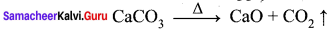Question 14.
How will you manage sulphur dioxide produced during roasting process?
The sulphur dioxide produced during roasting process is harmful to the environment. In modem metallurgical factories, this by product is trapped and converted into sulphuric acid to avoid air pollution.

Question 15.
What is smelting?
Smelting is a process of reducing the roasted metallic oxide to metal in molten condition. In this process, impurities are removed by the addition of flux as slag.

Question 16.
Explain Auto reduction.
Simple roasting of some of the ores give the crude metal. In such cases, the use of reducing agents is not necessary. For example, mercury is obtained by roasting of its ore cinnabar (HgS)
HgS (s) + O2(g) → Hg (l) + SO2 $$\uparrow$$

Question 17.
What is Ellingham diagram?
The graphical representation of variation of the standard Gibbs free energy of reaction for the formation of various metal oxides with temperature is called Ellingham diagram.

Question 18.
CO is more stable at higher temperature. Why?
In the Ellingham diagram, the formation of carbon monoxide is a straight line with negative slope. In this case ∆S is positive as 2 moles of CO gas is formed by the consumption of one mole of oxygen. Hence, CO is more stable at higher temperature.

Question 19.
Ag2(g)O and HgO are unstable at moderate temperature and they will decompose on heating even in the absence of a reducing agent. Why?
Ellingham diagram for the formation of Ag2(g)O and HgO is at upper part of the diagram and their decomposition temperatures are 600 and 700 K respectively. It indicates that these oxides are unstable at moderate temperatures and will decompose on heating even in the absence of a reducing agent.

Question 20.
What is refining process?
Generally the metal extracted from its ore contains some impurities such as unreacted oxide ore, other metals, nonmetal etc. Removal of such impurities associated with the isolated crude metal is called refining process.

Question 21.
List out the common refining methods.

1. Distillation
2. Liquation
3. Electrolytic refining
4. Zone refining
5. Vapour phase method

Question 22.
Explain Distillation process with suitable example.
This method is employed for low boiling volatile metals like zinc (boiling point 1180 K) and mercury (630 K). In this method, the impure metal is heated to evaporate and the vapours are condensed to get pure metal.

Question 23.
Write the applications of copper.

1. Copper is the first metal used by the humans and extended use of its alloy bronze resulted in a new era, Bronze age.
2. Copper is used for making coins and ornaments along with gold and other metals.
3. Copper and its alloys are used for making wires, water pipes and other electrical parts.

Question 24.
Why aluminium cannot be extracted by reducing alumina with carbon?
Alumina (Al2O3) cannot be reduced by using carbon because aluminum has more affinity for oxygen than carbon. Therefore, aluminium cannot be extracted by reducing alumina with carbon.

Question 25.
Write the names of two ores of copper.
Main ores of copper are:

1. Copper pyrites – CuFeS2
2. Copper glance – Cu2S
3. Malachite – CuCO3. Cu(OH)2

Question 26.
Explain the role of carbon monoxide in the purification of nickel?
During the purification of Nickel by Mond’s process, carbon monoxide (CO) is used to convert impure nickel to nickel carbonyl. Nickel carbonyl is an unstable compound. Heating to higher temperature decomposes it to give pure nickel.

Question 27.
ZnO can be reduced to the metal by heating with carbon but not Cr2O3. Justify your answer.
Carbon has more affinity for oxygen than zinc, whereas chromium has higher affinity for oxygen than zinc. Hence ZnO can be reduced to the metal by heating with carbon but not Cr2O3.

Question 28.
Name the method used for the refining of

1. Nickel
2. Zirconium

1. Mond’s process
2. Van Arkel’s method

Question 29.
Give one example for

1. Acidic flux
2. Basic flux

1. Acidic flux: SiO2
2. Basic flux: CaCO3, MgCO3

Question 30.
Why is the reduction of a metal oxide easier if the metal formed is in liquid state at the temperature of reduction?
The entropy is higher if the metal is in liquid state than when it is in solid state. The value of entropy change (∆S) of the reduction process is more on +ve side when the metal formed is in liquid state and the metal oxide being reduced is in solid state. Thus, the value of ∆G° becomes more on negative side and the reduction becomes easier.

Samacheer Kalvi 12th Chemistry Metallurgy 3 Mark Questions and Answers

Question 1.
All ores are minerals, but all minerals are not ores. Explain.
A naturally occurring substance obtained by mining which contains the metal in free state or in the form of compounds is called a mineral. In most of the minerals, the metal of interest is present only in small amounts and some of them contains a reasonable percentage of metal. Such minerals that contains a high percentage of metal, from which it can be extracted conveniently and economically are called ores. Hence all ores are minerals but all minerals are not ores.

Question 2.
Bauxite is an ore of aluminium while china clay is not. Why?
Bauxite and china clay, both are minerals of aluminium. However, aluminium can be commercially extracted from bauxite while extraction from china clay is not a profitable one. Hence the mineral, bauxite is an ore of aluminium while china clay is not.

Question 3.
Explain Gravity separation process or Hydraulic washing process?
[OR]
How will you concentrate oxide ores?
[OR]
Explain the suitable method to concentrate hematite and tinstone ores.
In this method, the ore having high specific gravity is separated from the gangue that has low specific gravity by simply washing with running water. Ore is crushed to a finely powdered form and treated with rapidly flowing current of water. During this process the lighter gangue particles are washed away by the running water. This method is generally applied to concentrate the native ore such as gold and oxide ores such as hematite (Fe2O3), tin stone (SnO2) etc.

Question 4.
Write a notes on alkali leaching process?
In this method, the ore is treated with aqueous alkali to form a soluble complex. For example, bauxite, an important ore of aluminium is heated with a solution of sodium hydroxide or sodium carbonate in the temperature range 470 – 520 K at 35 atm to form soluble sodium meta- aluminate leaving behind the impurities, iron oxide and titanium oxide.
Al2O3 (S) + 2NaOH (aq) + 3H2O (l) → 2Na[Al(OH)4] (aq)

The hot solution is decanted, cooled, and diluted. This solution is neutralised by passing CO2 gas, to the form hydrated Al2O2 precipitate.
2Na[Al(OH)4](aq) + CO2(g) → Al2O3. xH2O(s) + 2NaHCO3 (aq)
The precipitate is filtered off and heated around 1670 K to get pure alumina Al2O3.

Question 5.
Complete the following reaction.

1. ZnS + O2 $$\underrightarrow { \Delta }$$ ?
2. P4+ O2 $$\underrightarrow { \Delta }$$?
3. CaCO3 $$\underrightarrow { \Delta }$$ ?

1. 2ZnS + 3O2 $$\underrightarrow { \Delta }$$ 2ZnO + 2SO2 $$\uparrow$$
2. P4+ 5O2 $$\underrightarrow { \Delta }$$ P4O10 $$\uparrow$$
3. CaCO3 $$\underrightarrow { \Delta }$$ CaO + C02 $$\uparrow$$

Question 6.
Explain Aluminothermic process.
Metallic oxides such as Cr2O3 can be reduced by an aluminothermic process. In this process, the metal oxide is mixed with aluminium powder and placed in a fire clay crucible. To initiate the reduction process, an ignition mixture (usually magneisium and barium peroxide) is used.
BaO2 + Mg → BaO + MgO

During the above reaction a large amount of heat is evolved (temperature upto 2400°C, is generated and the reaction enthalpy is – 852 kJ mol ) which facilitates the reduction of Cr2O3 by aluminium power.
Cr2O3 + 2Al $$\underrightarrow { \Delta }$$ 2Cr + Al2O3

Question 7.
Why aluminium can be used as a reducing agent for the reduction of chronic oxide?
Ellingham diagram is used to predict thermodynamic feasibility of reduction of oxides of one metal by another metal. Any metal can reduce the oxides of other metals that are located above it in the diagram. For example, in the Ellingham diagram, for the fonnation of chromium oxide lies above that of the aluminium, meaning that Al2O3, is more stable than Cr2O3. Hence aluminium can be used as a reducing agent for the reduction of chromic oxide. However, it cannot be used to reduce the oxides of magnesium and calcium which occupy lower position than aluminium oxide.

Question 8.
Write notes on liquation.
Liquation:
This method, is employed to remove the impurities with high melting points from metals having relatively low melting points such a stin(Sb;mp=904K), lead(Pb;mp=600K), mercury(Hg; mp = 234 K), and bismuth (Bi; mp = 545K). In this process, the crude metal is heated to form fusible liquid and allowed to flow on a sloping surface.

The impure metal is placed on sloping hearth of a reverberatory furnace and it is heated just above the melting point of the metal in the absence of air, the molten pure metal flows down and the impurities are left behind. The molten metal is collected and solidified.

Question 9.
Write the application of Iron (Fe).
1. Iron is one of the most useful metals and its alloys are used everywhere including bridges, electricity pylons, bicycle chains, cutting tools and rifle barrels.

2. Cast iron is used to make pipes, valves and pumps stoves etc.

3. Magnets can be made of iron and its alloys and compounds.

4. An important alloy of iron is stainless steel, and it is very resistant to corrosion. It is used in architecture, bearings, cutlery, surgical instruments and jewellery. Nickel steel is used for making cables, automobiles and aeroplane parts. Chrome steels are used for manufacturing cutting tools and curshing machines

Question 10.
Mention the uses of Gold (Au).
1. Gold, one of the expensive and precious metals. It is used for coinage, and has been used as standard for monetary systems in some countries.

2. It is used extensively in jewellery in its alloy form with copper. It is also used in electroplating to cover other metals with a thin layer of gold which are used in watches, artificial limb joints, cheap jewellery, dental fillings and electrical connectors.

3. Gold nanoparticles are also used for increasing the efficiency of solar cells and also used an catalysts.

Question 11.
The extraction of Au by leaching with NaCN involves both oxidation and reduction. Justify giving equation.

• 4Au (s) + 8CN(aq) + 2H2O2(aq) + O2(g) → 4[Au(CN)2] (aq) + 4OH(aq)
• 2[Au(CN)2](aq) + Zn(s) → 2Au(s)+ [Zn(CN)4]-2(aq)

In the first reaction Au changes into Au+, i.e. its oxidation takes place. In the second reaction:
Au+ → Au°
(i.e.) reduction takes place.

Question 12.
Account for the following facts:

1. The reduction of a metal oxide is easier if the metal formed is in liquid state at the temperature of reduction.
2. The reduction of Cr2O3 with Al is thermodynamically feasible, yet it does not occur at room temperature.
3. Pine oil is used in froth floatation method.

1. In liquid state entropy is higher than the solid form, this makes ∆fG more negative.
2. By increasing the temperature, fraction of activated molecules increases, which helps in crossing over the energy barrier.
3. Pine oil enhances the non-wetting property of the ore particles and also acts as the froth collector.

Question 13.
Write the chemical reactions for purification of Zirconium by Van Arkel’s process.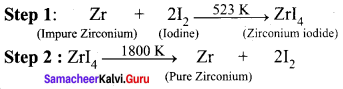Samacheer Kalvi 12th Chemistry Metallurgy 5 Mark Questions and Answers

Question 1.
Explain froth floatation process.
(Or)
How will you concentrate sulphide ores?
(Or)
Explain-the concentration of copper pyrites and galena ores.
Froth flotation:
This method is commonly used to concentrate sulphide ores such as galena (PbS), zinc blende (ZnS) etc. In this method, the metallic ore particles which are preferentially wetted by oil can be separated from gangue. In this method, the crushed ore is suspended in water and mixed with frothing agent such as pine oil, eucalyptus oil etc. A small quantity of sodium ethyl xanthate which acts as a collector is also added. A froth is generated by blowing air through this mixture.

The collector molecules attach to the ore particle and make them water repellent. As a result, ore particles, wetted by the oil, rise to the surface along with the froth. The froth is skimmed off and dried to recover the concentrated ore. The gangue particles that are preferentially wetted by water settle at the bottom.

When a sulphide ore of a metal of interest contains other metal sulphides as impurities, depressing agents such as sodium cyanide, sodium carbonate etc are used to selectively prevent other metal sulphides from coming to the froth. For example, when impurities such as ZnS is present in galena (PbS), sodium cyanide (NaCN) is added to depresses the flotation property of ZnS by forming a layer of zinc complex Na2[Zn(CN)4] on the surface of zinc sulphide.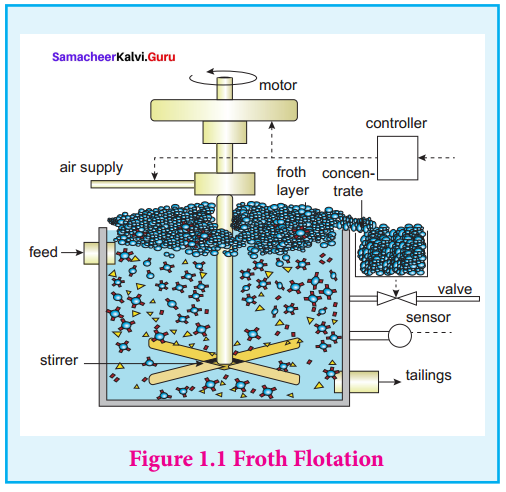Question 2.
Discuss the magnetic separation process.
(Or)
How will you separate magnetic ores from non-magnetic ores?
Magnetic separation:
This method is applicable to ferromagnetic ores and it is based on the difference in the magnetic properties of the ore and the impurities. For example tin stone can be separated from the wolframite impurities which is magnetic. Similarly, ores such as chromite, pyrolusite having magnetic property can be removed from the non magnetic siliceous impurities.

The crushed ore is poured on to an electromagnetic separator consisting of a belt moving over two rollers of which one is magnetic. The magnetic part of the ore is attracted towards the magnet and falls as a heap close to the magnetic region while the nonmagnetic part falls away from it as shown in the figure.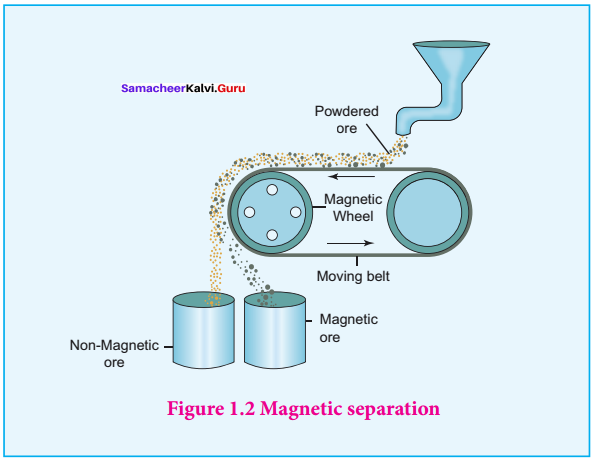Question 3.
Write a note on thermodynamic principle of metallurgy.
Thermodynamic principle of metallurgy:
The extraction of metals from their oxides can be carried out by using different reducing agents. For example, consider the reduction of a metal
oxide MxOy.
$$\frac { 2 }{ y }$$MxOy(s) → $$\frac { 2x }{ y }$$M(s) + O2(g) ……………..(1)

The above reduction may be carried out with carbon. In this case, the reducing agent carbon may be oxidised to either CO or CO2.

• C + O2 → CO2(g) ……….(2)
• 2C + O2 → 2CO(g) …………(3)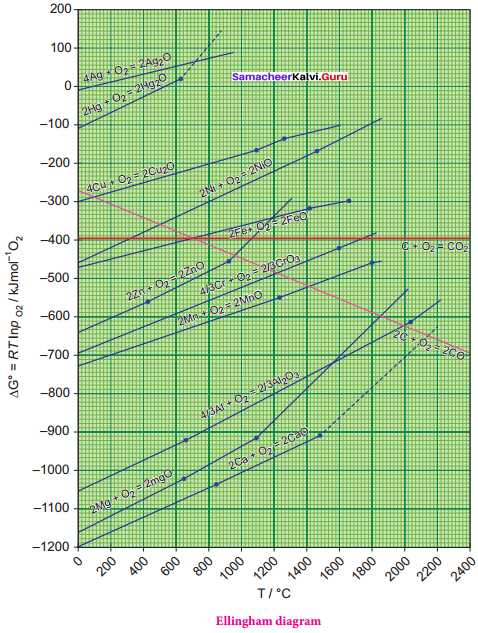If carbon monoxide is used as a reducing agent, it is oxidised to CO2 as follows,
2CO + O2 → 2CO2(g)  ……………(4)

A suitable reducing agent is selected based on the thermodynamic considerations. We know that for a spontaneous reaction, the change in free energy (AG) should be negative. Therefore, thermodynamically, the reduction of metal oxide [equation (1)] with a given reducing agent [Equation (2), (3) or (4)] can occur if the free energy change for the coupled reaction. [Equations (1) & (2), (1) & (3) or (1) & (4)] is negative. Hence, the reducing agent is selected in such a way that it provides a large negative AG value for the coupled reaction.

Ellingham diagram:
The change in Gibbs free energy (∆G) for a reaction is given by the expression.
∆G = ∆H – T∆S ……….(1)
where, ∆H is the enthalpy change , T the temperature in kelvin and ∆S the entropy change. For an equilibrium process, ∆G° can be calculated using the equilibrium constant by the following expression ∆G° = – RT lnKp

Harold Ellingham used the above relationship to calculate the ∆G° values at various temperatures for the reduction of metal oxides by treating the reduction as an equilibrium process. He has drawn a plot by considering the temperature in the x-axis and the standard free energy change for the formation of metal oxide in y-axis. The resultant plot is a straight line with ∆S as slope and ∆H as y-intercept. The graphical representation of variation of the standard Gibbs free energy of reaction for the formation of various metal oxides with temperature is called Ellingham diagram.

Question 4.
Explain the observations from the Ellingham diagram.
1. For most of the metal oxide formation, the slope is positive. It can be explained as follows. Oxygen gas is consumed during the formation of metal oxides which results in the decrease in randomness. Hence, ∆S becomes negative and it makes the term, T∆S positive in the straight line equation.

2. The graph for the formation of carbon monoxide is a straight line with negative slope. In this case ∆S is positive as 2 moles of CO gas is formed by the consumption of one mole of oxygen gas. It indicates that CO is more stable at higher temperature.

3. As the temperature increases, generally ∆G value for the formation of the metal oxide become less negative and becomes zero at a particular temperature. Below this temperature, ∆G is negative and the oxide is stable and above this temperature ∆G is positive. This general trend suggests that metal oxides become less stable at higher temperature and their decomposition becomes easier.

4. There is a sudden change in the slope at a particular temperature for some metal oxides like MgO, HgO. This is due to the phase transition (melting or evaporation).

Question 5.
Discuss.the applications of the Ellingham diagram:
Ellingham diagram helps us to select a suitable reducing agent and appropriate temperature range for reduction. The reduction of a metal oxide to its metal can be considered as a competition between the element used for reduction and the metal to combine with oxygen.

If the metal oxide is more stable, then oxygen remains with the metal and if the oxide of element used for reduction is more stable, then the oxygen from the metal oxide combines with elements used for the reduction. From the Ellingham diagram, we can infer the relative stability of different metal oxides at a given temperature.

1. Ellingham diagram for the formation of Ag2O and HgO is at upper part of the diagram and their decomposition temperatures are 600 and 700 K respectively. It indicates that these oxides are unstable at moderate temperatures and will decompose on heating even in the absence of a reducing agent.

2. Ellingham diagram is used to predict thermodynamic feasibility of reduction of oxides of one metal by another metal. Any metal can reduce the oxides of other metals that are located above it in the diagram. For example, in the Ellingham diagram, for the formation of chromium oxide lies above that of the aluminium, meaning that Al2O3 is more stable than Cr2O3. Hence aluminium can be used as a reducing agent for the reduction of chromic oxide. However, it cannot be used to reduce the oxides of magnesium and calcium which occupy lower position than aluminium oxide.

3. The carbon line cuts across the lines of many metal oxides and hence it can reduce all those metal oxides at sufficiently high temperature. Let us analyse the thermodynamically favourable conditions for the reduction of iron oxide by carbon. Ellingham diagram for the formation of FeO and CO intersects around 1000 K.

Below this temperature the carbon line lies above the iron line which indicates that FeO is more stable than CO and hence at this temperature range, the reduction is not thermodynamically feasible. However, above 1000 K carbon line lies below the iron line and hence, we can use coke as reducing agent above this temperature. The following free energy calculation also confirm that the reduction is thermodynamically favoured.

From the Ellingham Diagram at 1500 K:

• 2Fe(s) + O2(g) → 2FeO(g)
• 2C(s) + O2(g) → 2CO(g)
• ∆G1 = – 350 kJ mol-1 …………(5)
• ∆G2 = – 480 kJ mol-1 ………….(6)

Reverse the reaction (1)

• 2FeO(s) → 2Fe(s) + O2(g)
• – ∆G1= +350 kJ mol-1 ………. (7)

Now couple the reactions (2) and (3)

• 2FeO(s) + 2C 2Fe (l,s) + 2CO(g)
• ∆G3 = -130 kJ mol-1 ……………. (8)

The standard free energy change for the reduction of one mole of FeO is:
∆G3/2 = -65 kJ mol-1.

Question 6.
Explain the method to purify Titanium metal.
[OR]
Explain Van-Arkel method for refining Titanium.
[OR]
How will you purify metals by using iodine?
This method is based on the thermal decomposition of metal compounds which lead to the formation of pure metals. Titanium and zirconium can be purified using this method. For example, the impure titanium metal is heated in an evacuated vessel with iodine at a temperature of 550 K to form the volatile titanium tetra-iodide.(TiI4). The impurities are left behind, as they do not react with iodine.
Ti(s) + 2I2(s) → TiI4 (vapour)

The volatile titanium tetraiodide vapour is passed over a tungsten filament at a temperature around 1800 K. The titanium tetraiodide is decomposed and pure titanium is deposited on the filament. The iodine is reused.
Til4 (vapour) → Ti(s)+ 2I2(s)

Question 7.
Mention the applications of Aluminium.
Aluminium is the most abundant metal and is a good conductor of electricity and heat. It also resists corrosion. The following are some of its applications.

1. Many heat exchangers/sinks and our day to day cooking vessels are made of aluminium.
2. It is used as wraps (aluminium foils) and is used in packing materials for food items,
3. Aluminium is not very strong, However, its alloys with copper, manganese, magnesium and silicon are light weight and strong and they are used in design of aeroplanes and other forms of transport.
4. As Aluminium shows high resistance to corrosion, it is used in the design of chemical reactors, medical equipments,refrigeration units and gas pipelines.
5. Aluminium is a good electrical conductor and cheap, hence used in electrical overhead electric cables with steel core for strength.

Question 8.
Complete the following reactions,

1. Cr2O3 + A1 $$\underrightarrow { \Delta }$$ ?
2. B2O2 + Na $$\underrightarrow { \Delta }$$ ?
3. ThO2 + Ca $$\underrightarrow { \Delta }$$ ?
4. Mn3O4 + C $$\underrightarrow { \Delta }$$ ?
5. Ag2O + H2 $$\underrightarrow { \Delta }$$ ?

1. Cr2O3 + 2Al $$\underrightarrow { \Delta }$$ 2Cr +Al2O3
2. B2O3 + 6Na $$\underrightarrow { \Delta }$$ 2B +3Na2O
3. ThO2 + 2Ca $$\underrightarrow { 1250K }$$ Th + 2CaO
4. Mn3O4 + 4C $$\underrightarrow { \Delta }$$ 3Mn + 4CO
5. Ag2O + H2 $$\underrightarrow { \Delta }$$ 2Ag + H2O

Common Errors and its Rectifications

Common Errors:

1. In balancing inorganic reactions, they may struggle.
2. Ores formula they may forget.
3. In metallurgy, the steps are very important.

Rectifications:

1. Students are advised to balance first the negative charged atoms like oxygen, chlorine in the equation.
2. Simple way is to remember one oxide ore (or) one sulphide ore from which the metal is extracted.
3. Students should practice to write steps headings first along with explanation.

Hope you love the Samacheer Kalvi 12th Chemistry Chapter Wise Material. Clearly understand the deep concept of Chemistry learning with the help of Tamilnadu State Board 12th Chemistry Chapter 1 Metallurgy Questions and Answers PDF. Refer your friends to and bookmark our website for instant updates. Also, keep in touch with us using the comment section.